# Floyd Warshall Algorithm | C Programming | Factsprime

## How Floyd-Warshall Algorithm Works?

Let the given graph be: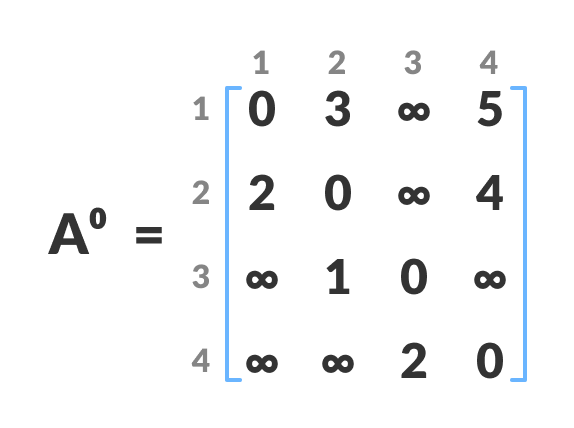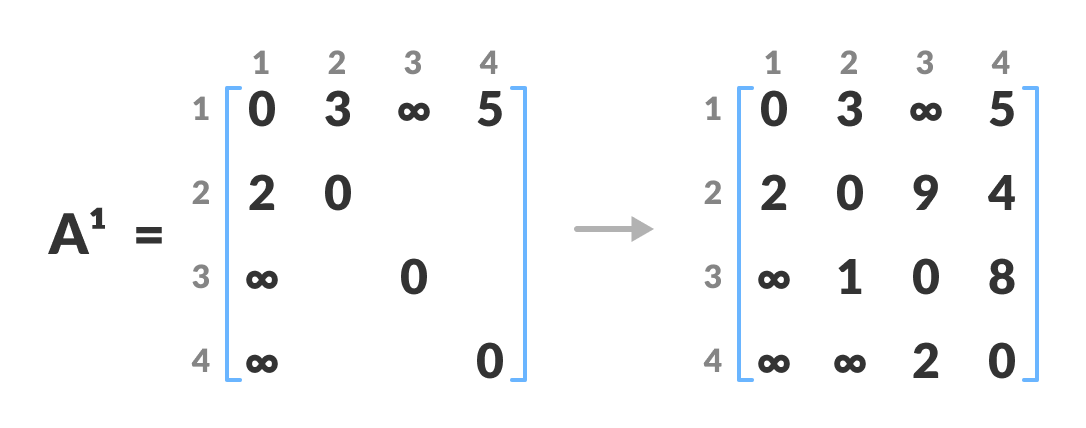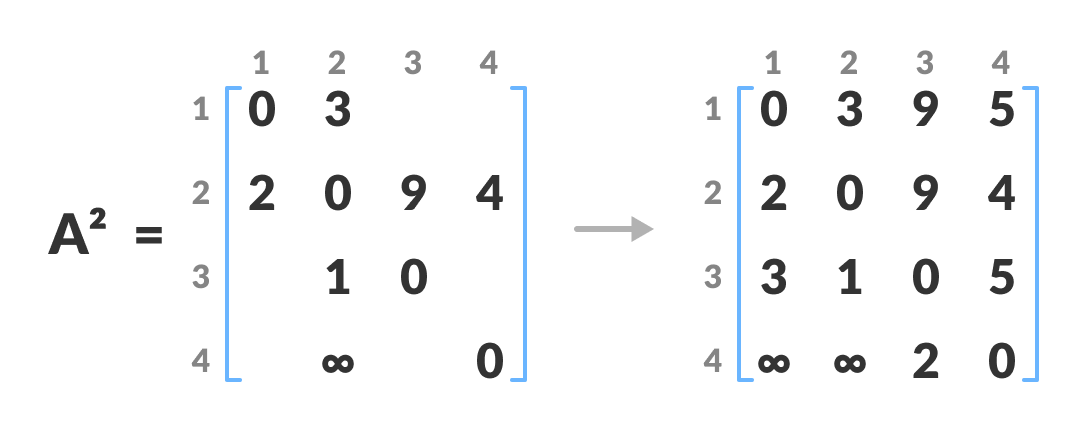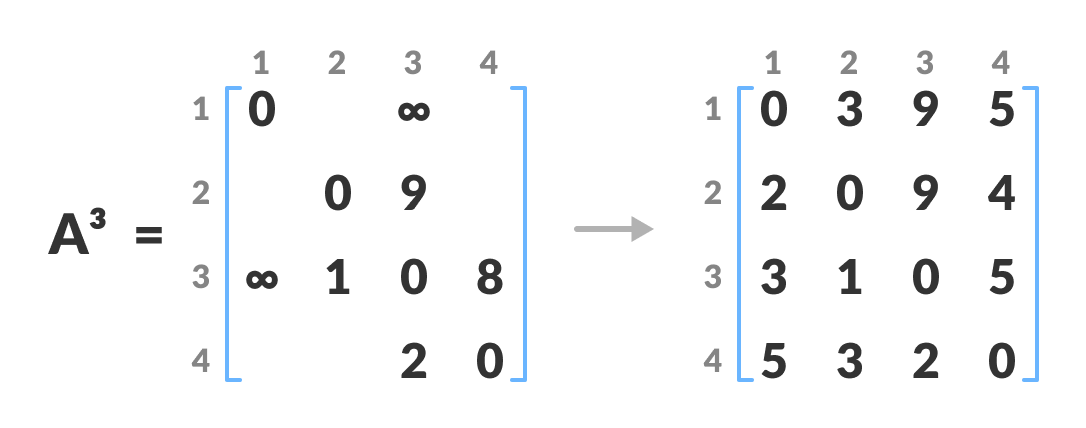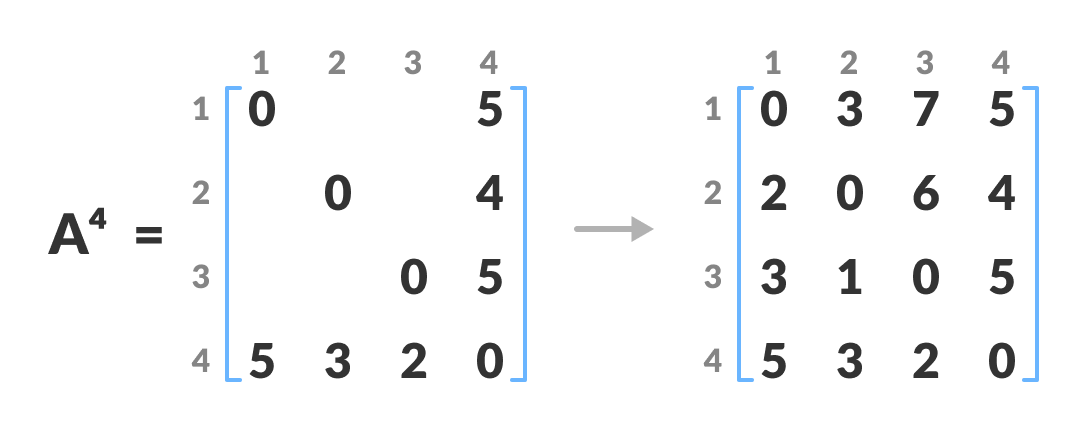CODE:

```#include<stdio.h>
#include<limits.h>

#define V 4 // Number of vertices

void floyd_warshall(int dist[V][V]) {
int i, j, k;

// Compute shortest paths for all pairs of vertices
for (k = 0; k < V; k++) {
for (i = 0; i < V; i++) {
for (j = 0; j < V; j++) {
if (dist[i][k] != INT_MAX && dist[k][j] != INT_MAX &&
dist[i][k] + dist[k][j] < dist[i][j]) {
dist[i][j] = dist[i][k] + dist[k][j];
}
}
}
}

// Print the shortest distances
printf("Shortest distances between every pair of vertices:\n");
for (i = 0; i < V; i++) {
for (j = 0; j < V; j++) {
if (dist[i][j] == INT_MAX) {
printf("INF ");
} else {
printf("%d   ", dist[i][j]);
}
}
printf("\n");
}
}

int main() {
int graph[V][V] = {{0, 5, INT_MAX, 10},
{INT_MAX, 0, 3, INT_MAX},
{INT_MAX, INT_MAX, 0, 1},
{INT_MAX, INT_MAX, INT_MAX, 0}};

floyd_warshall(graph);

return 0;
}

```

OUTPUT

```Shortest distances between every pair of vertices:
0   5   8   9
INF 0   3   4
INF INF 0   1
INF INF INF 0

Process returned 0 (0x0)   execution time : 0.038 s
Press any key to continue.```

Please find some more codes of Loops, Condition Statements, 1D Arrays, 2D Arrays, Strings, Pointers, Data Structures, Files, Linked lists, Stacks, Queues, Binary Trees, MISC, Solved model question papers & Hacker Rank all solutions on the below page:

Top 100+ C Programming codes – KLE Technological University